# Custom formulas

You can create a new value out of existing field values, similar to what you can do in a spreadsheet. There are three options for formulas:

## Roll-up

A roll-up is the sum of different field values.

Example

A roll-up formula is created using Insights and Votes as inputs.

Insights

Roll-up

5

8

13

## Weighted Score

A weighted score helps understand the value of an idea relative to other ideas in the project using normalization.

### What is normalization?

Normalization is an operation over the field values across all ideas:

• 0 is the lower value, or we use the lower one if there is a negative value.

• The highest field value is 100.

• All values are then plotted based on their relative value between those two.

Example

Ideas

Rating

Normalized score

Idea 1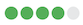100

Idea 2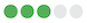75

Idea 3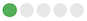25

## Formula

In the weighted formula:

• Each field (input) is normalized in isolation.

• The weights are applied.

• The result of this operation is normalized.

Note: When there are both positive and negative values, there is an additional operation to divide the sums of positive inputs by the sum of negative inputs.

Previously, the calculation was a normalized sum of all normalized field value multiplied by their weights: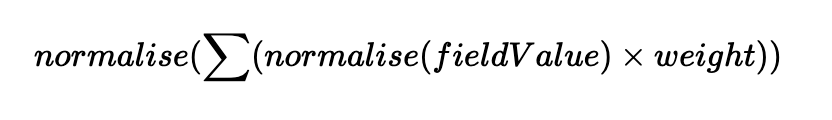Now we have 2 cases:

1. We only have positive weights defined, then we fallback to the previous formula (the one displayed above).

2. We have at least one negative weight, and the calculation is done by dividing the normalized sum of all positive input’s normalized field values multiplied by their weights by the normalized sum of all negative input’s normalized field values multiplied by their weights: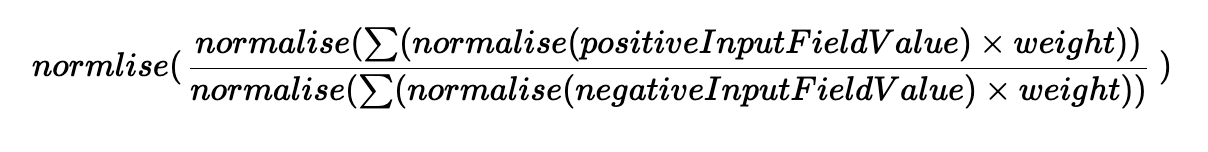Example:

Normalize ( Normalize ( Positive1 ) * weightP1 +  ...  + Normalize ( PositiveX ) * weightPX)

Normalize ( Normalize ( Negative1 ) * weightN1 +  ...  + Normalize ( PositiveX ) * weightNX)

If you put everything in the positive inputs section and use a negative number in the positive input section, it will normalize by adding everything, including the negative number.

You can write your own formula using a simple expression language:

Data Type

Example

Notes

Number

10 , 3.5

Scientific notation and negative numbers aren’t supported.

Field

{Goal impact}

Internal spaces are preserved, but leading and trailing spaces are removed.

Operator

Example

Notes

+

{Goal Impact} + 10

-

{Goal Impact} - {Effort}

Subtraction

*

{Num data points} * 3

Multiplication

/

1 / {Effort}

Division